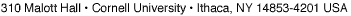## MATH 7110: Seminar in Analysis: Riemannian Geometry and Geometric Analysis (fall 2008)

Instructor: Xiaodong Cao

This is a topic course of Riemannian geometry and geometric analysis. It will be a continuation of MATH 662. We will cover the following topics: comparison theorems and gradient estimates, harmonic functions on manifolds with negative curvature, eigenvalue problems, heat kernel on Riemannian manifolds, conformal deformation of scalar curvatures, etc. We will use Lectures on differential geometry by Schoen and Yau.